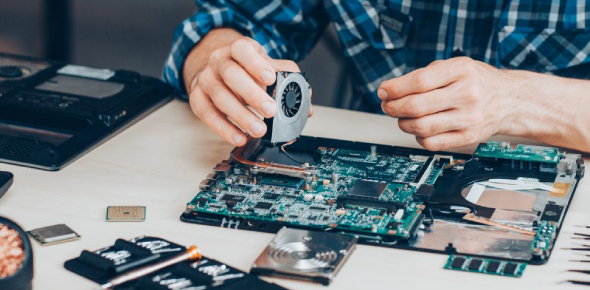Computer Hardware Knowledge Trivia: MCQ Quiz

25 Questions | Total Attempts: 176SettingsWelcome to the Computer Hardware Knowledge MCQ Quiz. Computer components such as the CPU, motherboard, computer case, RAM, and drive are the computer's core pieces. They determine the capabilities and features of computing as well as it's power and processing speeds. Take on this quiz and put your knowledge of computer hardware to the test.

• 1.
Resolution of display card is
• A.

The number of pixels

• B.

The number of colours

• C.

The amount of memory

• D.

The speed of CPU

• 2.
External modem requires a/an __________ to get powered on
• A.

• B.

• C.

• D.

Data cable

• 3.
The output of a blue color wire in an SMPS is
• A.

-12v DC

• B.

13v DC

• C.

22v DC

• D.

23v DC

• 4.
In a colour monitor, 1 pixel is represented by ___________.
• A.

• B.

Nibble

• C.

Both (1) and (2)

• D.

Btye

• 5.
In a DVD- ROM, tracks are placed ________ mm apart.
• A.

0.74

• B.

10

• C.

164

• D.

74

• 6.
The output of red colour wire in a SMPS is
• A.

+5v DC

• B.

+12v DC

• C.

-5v DC

• D.

-3.3v DC

• 7.
Which IRQ number is used by floppy disk controller?
• A.

1

• B.

5

• C.

6

• D.

8

• 8.
Which of the following is the type of logical memory?
• A.

RAM

• B.

EEPROM

• C.

Pen drive

• D.

Extended Memory

• 9.
To which port of computer system can pen drive connect?
• A.

USB

• B.

PS/2 port

• C.

Serial port

• D.

Parallel port

• 10.
Which of the following memories is used by a pen drive?
• A.

Flash memory

• B.

Cache memory

• C.

Physical memory

• D.

RAM

• 11.
Which power connector is used to supply power to the motherboard?
• A.

PCI

• B.

AGP

• C.

PS/2

• D.

ATX

• 12.
How many pins does a DIMM package contain?
• A.

144 pins

• B.

168 pins

• C.

172 pins

• D.

72 pins

• 13.
Baby AT motherboard supports maximum of _____ slots.
• A.

7

• B.

8

• C.

2

• D.

4

• 14.
Which of the following is also known as a printed circuit board in the computer system?
• A.

RAM

• B.

Micro-processor

• C.

Motherboard

• D.

Jumper

• 15.
_______________ store(s) the time and settings of a system.
• A.

Memory slots

• B.

CPU socket

• C.

CMOS chip

• D.

BIOS chip

• 16.
IRQ 8 is assigned to ________.
• A.

Keyboard

• B.

Network card

• C.

Sound Card

• D.

Real time clock

• 17.
1 nibble is equivalent to
• A.

4 bits

• B.

8 bits

• C.

32 bits

• D.

None of these

• 18.
With the help of which scanner can you scan photo slides?
• A.

Slide scanner

• B.

Drum scanner

• C.

Flat bed scanner

• D.

Sheet fed scanner

• 19.
The scanning surface of a scanner is made up of _________.
• A.

Plastic

• B.

Wood

• C.

Ceramic

• D.

Glass

• 20.
You can use the ______________ scanner for scanning large individual sheets of paper.
• A.

Slide

• B.

Sheet

• C.

Sheet fed

• D.

Drum

• 21.
CD-ROM stands for
• A.

• B.

• C.

• D.

• 22.
Which memory is termed as ' Destructive Memory'?
• A.

Primary Memory

• B.

Secondary Memory

• C.

Cache Memory

• D.

None of the above

• 23.
The number of bits that a memory card consists of is called its
• A.

Length

• B.

Byte

• C.

Word length

• D.

None of the above

• 24.
Which technology was used for storing a large amount of data in the Fourth Generation computers?
• A.

RAID

• B.

Hard disk

• C.

CD-Rom

• D.

None of the above

• 25.
Which section of the CPU selects and interprets program instruction and checks whether they are executed or not?
• A.

ALU section

• B.

Control Section

• C.

Memory

• D.

None of the above

Related TopicsBack to top